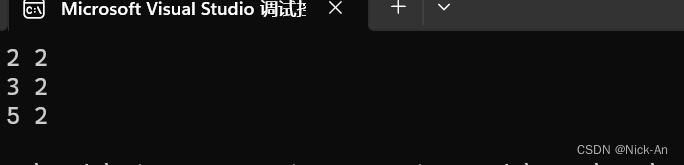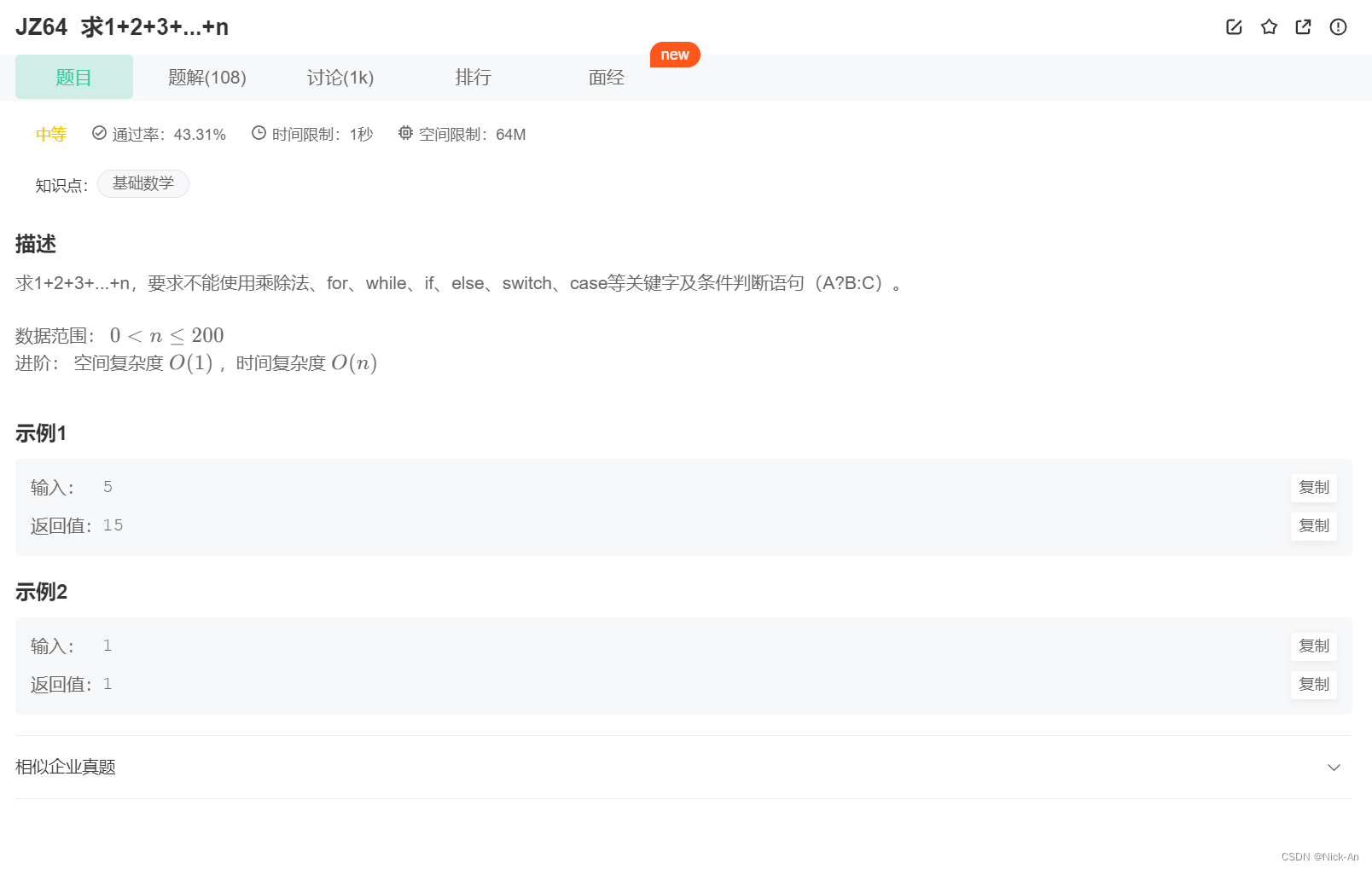## 类和对象——初始化列表

chatgpt/2023/9/26 13:56:21

explicit关键字

# 初始化列表

``````class Date
{
public:
//初始化列表—每个成员定义的地方Date(int year, int month, int day):_year(year) //成员变量会被赋值为括号内相应的值, _month(month), _day(day),_x(1){//_day=day;//此处只能写在中括号里，中括号外必须要有' () '去定义    }  //中括号内实现其他代码
private:int _year;int _month;int _day;const int _x=10;//const修饰的变量必须要在定义的时候初始化-后续不能修改，总之在类里必须有个地方进行赋值初始化
};``````

## 注意

1.缺省值是赋予初始化列表的

2.若初始化列表没有显示给值，就用这个缺省值

3.如果显示给值了，就不用这个缺省值(会先赋值缺省值,如果在列表里重新定义，就会重新赋值)

4.成员变量在类中声明次序就是其在初始化列表中的初始化顺序，与其在初始化列表中的先后 次序无关

## 单/多参数传入

``````//单参数
class A
{
public:A(int i):_a(i){cout << "a(int i)" << endl;}
private:int _a;
};
//多参数
class B
{
public:B(int b1, int b2)//explicit B(int b1, int b2):_b1(b1), _b2(b2){cout << "B(int b1, int b2)" << endl;}
private:int _b1;int _b2;
};int main()
{const A& ref = 2;B bb1 (1, 2);B bb2 = { 2,2 };const B& ref2 = { 3,3 };//相同空间用引用,需转换类型，加const修饰return 0;
}``````

# explicit关键字

``````class A
{
public://explicit A(int i)//在构造函数这里加上explicit，可以防止隐式转换——这样主函数的两个转换就不能使用了A(int i):_a(i){cout << "A(int i)" << endl;}A(const A& aa):_a(aa._a){cout << "A(const A& aa)" << endl;}~A(){cout << "Delete" << endl;}
private:int _a;
};int main()
{A aa1(1);/单参数构造函数的隐式类型转换//用2调用A构造函数，先生成一个临时对象，再用这个对象去拷贝构造aa2//编译器会优化，优化用2直接构造A aa2 = 2;//结果是直接构造，而不是先对2进行了拷贝构造，再把这个对象赋值给aa2//2会进行隐式类型转换，过程中会生成临时对象，具有常性，所以需要const去修饰const A& ref = 2;//2转换，生成了一个临时对象具有常性，需要用const来修饰return 0;
}``````

# 匿名函数

``    A aa6(6);//有名——生命周期在当前局部域A (7);//匿名——生命周期只在这一行//运行后发现的确调用了一次析构，说明出了这一行就会销毁//先定义再传入数据，代码量多SeqList s;s.PushBack(aa6);//用匿名对象传，直接放入想要的数据s.PushBack(A(8));``

``````class Solution {
public:int Sum_Solution(int n) {// ...cout << n << endl;return n;}
private:
};int main()
{Solution s1;s1.Sum_Solution(10);Solution().Sum_Solution(100);//这种方法无需定义一个对象，直接赋值，调用类里的函数。return 0;}``````

# Static成员

## 引入

``````//全局变量
int n=0;
int m=0;
class A
{A(){++n;++m;}A(const  A& t){++n;++m;}~A(){--m;}void Print(){cout<<m<<" "<<n<<endl;}
private:}
int main()
{A aa1;A aa2;cout << n << " " << m << endl;//共有情况,非privateA();cout << n << " " << m << endl;Func(aa1);cout << n << " " << m << endl;return 0;
}``````## 修正

``````class A
{
public:{//....}
//private://静态成员变量属于所有A对象，属于所有类/*int n=0 ;//创建int m=0 ;//正在使用 */err，因为这样的话，每个对象都会有一个m和n，但我们仅需要一个全局的，所有对象共有的static int n;static int m;}int A::m=0;
int A::n=0;``````

``````int main()
{A aa1;A aa2;cout << A::n << " " <<A:: m << endl;//共有情况,非privatecout << aa1.n << " " << aa1.m << endl;return 0;
}``````

## static定义的特点

### 1.static定义的变量处于静态区里，不参与类大小的计算

``sizeof(A);//———— 1``

``````int main()
{A* ptr = nullptr;cout << ptr->n << " " << ptr->m << endl;//为什么行得通？return 0;
}``````

' . '

## static静态成员函数

### 若用匿名函数

``````int main()
{A();//能否用匿名函数去打印？A();A::Print();return 0;
}``````

``static void Print(){//x++; //不能访问非静态，因为没有this指针cout << m <<" "<< n << endl;}``

### 静态成员函数特点

1.不具有this指针

2.不能访问非静态变量（仅能访问static定义的变量）

``````static int& GetM(){return m;}
++A::GetM();``````

## 例题（求1+2+3+...+n_牛客题霸_牛客网）

``````class Sum
{
public:Sum()//构造函数，开创一次就进入一次{_ret+=_i;_i++;}static int GetRet(){return _ret;}private:static int _ret;//用static修饰，这样可以保证所有对象共用这个_retstatic int _i;
};int Sum::_ret=0;
int Sum::_i=1;
class Solution
{
public:int Sum_solution(int n){Sum a[n]return Sum::GetRet();}
};``````

# 友元函数

``````class Date
{//友元声明——这样可以在外部访问私密对象//friend void operator<<(ostream& out, const Date& d);friend ostream& operator<<(ostream& out, const Date& d);friend istream& operator>>(istream& in, Date& d);
public:{//..}
private:{}
}``````

# 内部类

## 特点/定义

1.一个类定义在另一个类的内部

2.内部类是一个独立的类，不受限制，且是外部类的友元

``````class C
{
public:class D{public:void FuncD(){；}private:int _d;};
private:int _c;
};sizeof(C);//  ——4``````

3.内部类是外部类的友元，内部类可以读取外部类的变量

``````class C
{
public:class D{public:void FuncD(){C cc;cc._c = 1;//内部类D可以直接访问外部类C的私有成员变量}private:int _d;};void func(){D dd;}
private:int _c;
};``````

4.public不能去掉，这样的话D类就不属于C类的公共区域里，

``````int main()
{C::D dd1;//但是定义还是得用C类域内部的D类定义//若去掉public，那么D类不是公共的，就不能调用cc.func();//也不能调用，因为func是属于D类的，但是D类不公共return 0;
}``````

## 内部类实现循环

（求1+2+3+...+n_牛客题霸_牛客网 (nowcoder.com)）

``````class Solution {class Sum{public:Sum(){_ret+=_i;_i++;}};
public:int Sum_Solution(int n) { Sum a[n];return _ret;}
private:static int _ret;static int _i;
};int Solution::_ret=0;
int Solution::_i=1;``````

### pyspark 判断 Hive 表是否存在

Catalog.tableExists(tableName: str, dbName: Optional[str] None) → booltableName:表名 dbName&#xff1a;库名(可选) return&#xff1a;bool 值 spark SparkSession \.builder \.appName(tableExists) \.config(spark.num.executors, 6) \.config(spark.executor.memo…